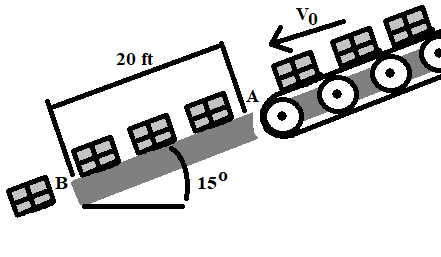# Boxes are transported by a conveyor belt with a velocity v0 to a fixed incline at A where they...

## Question:

Boxes are transported by a conveyor belt with a velocity {eq}v_0 {/eq} to a fixed incline at A where they slide and eventually fall off at B. Knowing that {eq}\mu_k {/eq} = 0.40, determine the velocity of the conveyor belt if the boxes are to have zero velocity at B.## Conservation of Energy:

The law of conservation of energy states that the total energy of an isolated system will remain conserved. It can be restated as that energy will only change its form but the total energy content of the system will remain will contact. For example in a spring block system with no friction at any point, the energy remains conserved. The kinetic energy of the block gets transferred to the spring and is stored in the spring in the form of potential energy. Thus, the total energy of the system only changes its form from kinetic to potential energy and vice-versa. If there was friction, then obvious observation would be that the block will stop. The energy is still not lost. The energy was used up in overcoming the friction and the energy got converted into heat and sound energy.

In the above situation, for the block to drop down with a zero initial velocity, the velocity at the edge of the platform must be zero. Which means all of the initial kinetic energy of the blocks must be consumed up in overcoming the friction by the time they cover 20ft.

Thus the kinetic friction acting along the direction of motion of blocks will be

{eq}f_k=\mu mgcos\theta\\ f_k=0.4 m(32.1714)cos15^\circ\\ {/eq}

The distance covered is 20ft. The initial kinetic energy is

{eq}\dfrac{1}{2}mv_o^2 {/eq}

By the time blocks reach the end, they will gain kinetic energy due to gravity. Thus we can write

{eq}E_{gravity}=mgsin\theta (d)\\ E_{gravity}=m(32.1714)sin(15^\circ)20 {/eq}

Thus we can write

{eq}20\times 0.4m(32.1714)cos15^\circ=\dfrac{1}{2}mv_o^2+m(32.1714)sin(15^\circ)20 {/eq}

That is just the work dissipated due to friction is equal to the total kinetic energy of the block that would have been there in the absence of any friction. Solving for {eq}v_o {/eq}, we get

{eq}v_o=\sqrt{20(32.1714)(0.4cos15^\circ-sin15^\circ)2}=12.81\ fts^{-1} {/eq}